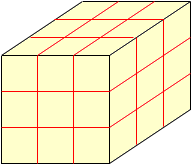Sender: Rhonda have 2.47 cubic yards. what could the dimensions, in feet, be to equal this? it does not have to be any specific size, just so the cube equals 2.47 cubic yards. Hi Rhonda, Imagine that you have a box that is a cube of length 1 yard on each side. Now fill it with cubes that are each 1 foot on each side as in the diagram. Since 1 yard is 3 feet you can see that it takes 3x3x3 = 27 one foot cubes to fill the box. Thus there are 27 cubic feet in one cubic yard.You have 2.47 cubic yards and hence you have 2.47x27 = 66.69 cubic feet. Cheers, Penny Go to Math Central